Processing ......FreeComputerBooks.com Free Computer, Mathematics, Technical Books and Lecture Notes, etc.

Mathematical Logic - Computability, Set Theory, Model Theory, Proof Theory, etc.Related Book Categories:
•A Friendly Introduction to Mathematical Logic (Chris Leary)

In this user-friendly book, readers with no previous study in the field are introduced to the basics of model theory, proof theory, and computability theory, leading to rigorous proofs of Gödel's First and Second Incompleteness Theorems.

•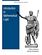Introduction to Mathematical Logic (Vilnis Detlovs, et al)

This book explores the principal topics of mathematical logic. It covers propositional logic, first-order logic, first-order number theory, axiomatic set theory, and the theory of computability. Discusses the major results of Gödel, Church, Kleene, Rosser, and Turing.

•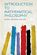Introduction to Mathematical Philosophy (Bertrand Russell)

Requiring neither prior knowledge of mathematics nor aptitude for mathematical symbolism, the book serves as essential reading for anyone interested in the intersection of mathematics and logic and in the development of analytic philosophy.

•What is Mathematics: Godel's Theorem and Around (K. Podnieks)

This accessible book gives a new, detailed and elementary explanation of the Godel incompleteness theorems and presents the Chaitin results and their relation to the da Costa-Doria results, which are given in full, but with no technicalities.

•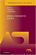Model-Theoretic Logics (Jon Barwise, et al)

This book brings together several directions of work in model theory between the late 1950s and early 1980s. It provides an introduction to the subject as a whole, as well as to the basic theory and examples. Many chapters can be read independently.

•A Problem Course in Mathematical Logic (Stefan Bilaniuk)

It is intended to serve as the text for an introduction to mathematical logic for undergraduates with some mathematical sophistication. It supplies definitions, statements of results, and problems, along with some explanations, examples, and hints.

•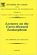Lectures on the Curry-Howard Isomorphism (M. H. Sorensen, et al)

This book gives an introduction to parts of proof theory and related aspects of type theory relevant for the Curry-Howard isomorphism. It can serve as an introduction to any or both of typed lambda-calculus and intuitionistic logic.

•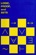Proof, Sets, and Logic (M. Randall Holmes)

Addressing the importance of constructing and understanding mathematical proofs, this book introduces key concepts from logic and set theory as well as the fundamental definitions of algebra to prepare readers for further study in the field of mathematics.

•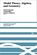Model Theory, Algebra, and Geometry (Deirdre Haskell, et al)

Tis book gives the necessary background for understanding both the model theory and the mathematics behind the apps, begins with an introduction to model theory, broadens into three components: pure model theory, geometry, and the model theory of fields.

•An Introduction to Set Theory (William A. R. Weiss)

This book covers the basics: relations, functions, orderings, finite, countable, and uncountable sets, and cardinal and ordinal numbers, gives students sufficient grounding in a rigorous approach to the revolutionary results of set theory as well as pleasure in being able to tackle significant problems that arise from the theory.

•Basic Model Theory (Kees Doets)

As the title indicates, this book introduces the reader to what is basic in model theory. A special feature is its use of the Ehrenfeucht game by which the reader is familiarised with the world of models.

•Fundamentals of Model Theory (William Weiss, et al)

This book is a concluding discussion focuses on the relationship between proofs and formal derivations, and the role proofs may play as part of a general theory of evidence.

•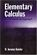Elementary Calculus: An Infinitesimal Approach (H. Jerome Keisler)

This book is concerned with the infinitesimal approach originally set forth by Newton and Leibnitz, using Non-standard analysis.

•A Concise Introduction to Mathematical Logic (W. Rautenberg)

This is a well-written introduction to the beautiful and coherent subject of mathematical logic. It contains classical material such as logical calculi, beginnings of model theory, and Goedel's incompleteness theorems, as well as some topics motivated by applications.

•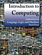Introduction to Computing: Language, Logic, and Machines

This book focuses on how to describe information processes by defining procedures, how to analyze the costs required to carry out a procedure, and the fundamental limits of what can and cannot be computed mechanically.

•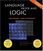Language, Proof and Logic (Jon Barwise, et al)

This book covers first-order language in a method appropriate for first and second courses in logic, and is specially useful to undergraduates of philosophy, computer science, mathematics, and linguistics.

•Mathematics in the Age of the Turing Machine (Thomas C. Hales)

Computers have rapidly become so pervasive in mathematics that future generations may look back to this day as a golden dawn. The article gives a survey of mathematical proofs that rely on computer calculations and formal proofs.

•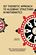Set Theoretic Approach to Algebraic Structures in Mathematics

This book brings out how sets in algebraic structures can be used to construct the most generalized algebraic structures, like set linear algebra / vector space, set ideals in groups and rings and semigroups, and topological set vector spaces.

•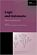Logic and Automata: History and Perspectives (Jorg Flum, et al)

This book gives a consolidated overview of the research results achieved in the theory of automata and logic, covers many different facets of logic and automata theory, emphasizing the connections to other disciplines such as games, algorithms, etc.

•Simply Logical: Intelligent Reasoning by Example (Peter Flach)

This book is an introduction to Prolog programming for artificial intelligence covering both basic and advanced AI material.

•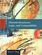Discrete Structures, Logic, and Computability (James L. Hein)

This book contains programming experiments that are designed to reinforce the learning of discrete mathematics, logic, and computability.

•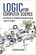Logic for Computer Science: Automatic Theorem Proving

This book introduces mathematical logic with an emphasis on proof theory and procedures for algorithmic construction of formal proofs. It is useful for the formalization of proofs and basics of automatic theorem proving.

•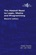The Haskell Road to Logic, Maths and Programming (Kees Doets)

The purpose of this book is to teach logic and mathematical reasoning in practice, and to connect logical reasoning with computer programming.

•Prolog Experiments in Discrete Math, Logic, and Computability

This book contains programming experiments that are designed to reinforce the learning of discrete mathematics, logic, and computability.

•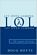Let Over Lambda - 50 Years of Lisp (Doug Hoyte)

This book is one of the most hardcore computer programming books out there. Starting with the fundamentals, it describes the most advanced features of the most advanced language: Common Lisp.

•Categories, Types, and Structures (Andrea Asperti, et al)

This book introduces category theory at a level appropriate for computer scientists and provides practical examples in the context of programming language design.

•Logic, Programming and Prolog, 2nd Edition (Ulf Nilsson, et al)

This book introduces major new developments in a continually evolving field and includes such topics as concurrency and equational and constraint logic programming.

•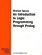An Introduction to Logic Programming Through Prolog (J. Spivey)

This is one of the few texts that combines three essential theses in the study of logic programming: logic, programming, and implementation.

•Inductive Logic Programming: Techniques and Applications

This book is an introduction to inductive logic programming (ILP), which aims at a formal framework as well as practical algorithms for inductively learning relational descriptions in the form of logic programs.

•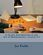A Gentle Introduction to the Art of Mathematics (Joseph E. Fields)

This book has chapters on Logic, Set theory, Relations and Cardinality interspersed with chapters on proofs - direct and indirect arguments, induction, combinatorial reasoning and "magic".

•Logics of Time and Computation (Robert Goldblatt)

This is a short but excellent introduction to modal, temporal, and dynamic logic, etc. It manages to cover, in highly readable style, the basic completeness, decidability, and expressability results in a variety of logics of the three kinds considered.

•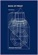Book of Proof, 2nd Edition (Richard H. Hammack)

This book is an introduction to the language and standard proof methods of mathematics. It lays a foundation for more theoretical courses such as topology, analysis and abstract algebra.

•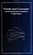Proofs and Concepts: The Fundamentals of Abstract Mathematics

This undergraduate textbook provides an introduction to proofs, logic, sets, functions, and other fundamental topics of abstract mathematics.

•Basic Concepts of Mathematics (Elias Zakon)

This book helps the student complete the transition from purely manipulative to rigorous mathematics, with topics that cover basic set theory, fields (with emphasis on the real numbers), a review of the geometry of three dimensions, and properties of linear spaces.

•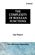The Complexity of Boolean Functions (Ingo Wegener)

Initially deals with the wee-known computation models, and goes on to special types of circuits, parallel computers, and branching programs.

•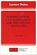Normalization, Cut-Elimination, and the Theory of Proofs

This book is a concluding discussion focuses on the relationship between proofs and formal derivations, and the role proofs may play as part of a general theory of evidence.

•Logical Reasoning (Bradley H. Dowden)

The goal of this book is to improve your logical-reasoning skills. Your most important critical thinking skill is your skill at making judgments-not snap judgments that occur in the blink of an eye, but those that require careful reasoning.

•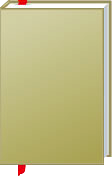Mathematical Logic - Computability, Set Theory, Model Theory, Proof Theory, etc.

This is the previous page of Mathematical Logic - Set Theory, Model Theory, Proof Theory, Computability, etc. we are in the processing to convert all the books there to the new page. Please check this page daily!!!

Book CategoriesAll CategoriesRecent BooksIT Research LibraryMiscellaneous Books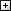Computer LanguagesComputer ScienceData Science/DatabasesElectronic EngineeringJava and Java EE (J2EE)Linux and UnixMathematicsMicrosoft and .NETMobile ComputingNetworking and CommunicationsSoftware EngineeringSpecial TopicsWeb Programming
Other Categories